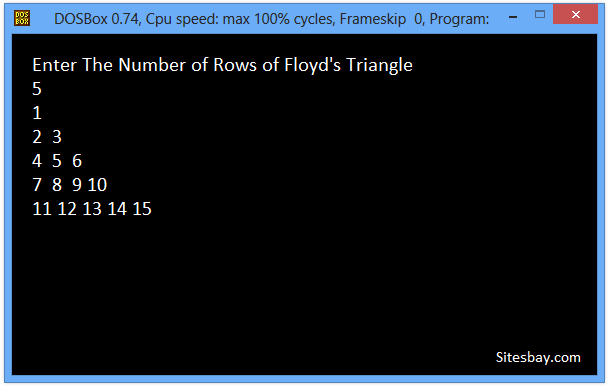# Print Floyd Triangle Program in C++

## C++ Program to Print Floyd Triangle

A Floyd's triangle is a right angled triangle of natural numbers arranged in increasing order from left to right such that Nth row contains N numbers.

For write this code we first take number of rows of Floyd's triangle as input from user and store it in a variable rows. Then using two for loops we will print N consecutive natural numbers in Nth row. Here outer for loop prints one row in every iteration where as inner for loop prints numbers of one row.

To understand below example, you have must knowledge of following C++ programming topics; Print Triangle of Stars in C++, for looping statements we need know For Loop in C++.

## Example

```1
2 3
4 5 6
7 8 9 10
11 12 13 14 15
16 17 18 19 20 21
```

## C++ Program to Transpose Matrix

```#include<iostream.h>
#include<conio.h>

void main()
{
int i, j, rows, counter;

cout <<"Enter The Number of Rows of Floyd's Triangle\n";
cin>>rows;

// Print Floyd's triangle
for (counter = 1, i = 1; i <= rows; i++) {
// Print ith row
for (j = 1; j <= i; j++) {
cout << counter++ << " ";
}
cout<<endl;
}
getch();
}
```

## Output

```Enter The Number of Rows of Floyd's Triangle
5
1
2  3
4  5  6
7  8  9 10
11 12 13 14 15
```WIFI CCTV Camera